15 November 2002

7 Coordinate Systems, Transformations and Units

7.1 Introduction

For all media, the SVG canvas describes "the space where the SVG content is rendered." The canvas is infinite for each dimension of the space, but rendering occurs relative to a finite rectangular region of the canvas. This finite rectangular region is called the SVG viewport. For visual media [ CSS2-VISUAL], the SVG viewport is the viewing area where the user sees the SVG content.

The size of the SVG viewport (i.e., its width and height) is determined by a negotiation process (see Establishing the size of the initial viewport) between the SVG document fragment and its parent (real or implicit). Once that negotiation process is completed, the SVG user agent is provided the following information:

• a number (usually an integer) that represents the width in "pixels" of the viewport
• a number (usually an integer) that represents the height in "pixels" of the viewport
• (highly desirable but not required) a real number value that indicates the size in real world units, such as millimeters, of a "pixel" (i.e., a px unit as defined in [ CSS2 lengths])

Using the above information, the SVG user agent determines the viewport, an initial viewport coordinate system and an initial user coordinate system such that the two coordinates systems are identical. Both coordinates systems are established such that the origin matches the origin of the viewport (for the root viewport, the viewport origin is at the top/left corner), and one unit in the initial coordinate system equals one "pixel" in the viewport. (See Initial coordinate system.) The viewport coordinate system is also called viewport space and the user coordinate system is also called user space.

Lengths in SVG can be specified as:

• (if no unit identifier is provided) values in user space -- for example, "15"
• (if a unit identifier is provided) a length expressed as an absolute or relative unit measure -- for example, "15mm" or "5em"

The supported length unit identifiers are: em, ex, px, pt, pc, cm, mm, in, and percentages.

A new user space (i.e., a new current coordinate system) can be established at any place within an SVG document fragment by specifying transformations in the form of transformation matrices or simple transformation operations such as rotation, skewing, scaling and translation. Establishing new user spaces via coordinate system transformations are fundamental operations to 2D graphics and represent the usual method of controlling the size, position, rotation and skew of graphic objects.

New viewports also can be established. By establishing a new viewport, you can redefine the meaning of percentages units and provide a new reference rectangle for "fitting" a graphic into a particular rectangular area. ("Fit" means that a given graphic is transformed in such a way that its bounding box in user space aligns exactly with the edges of a given viewport.)

7.2 The initial viewport

The SVG user agent negotiates with its parent user agent to determine the viewport into which the SVG user agent can render the document. In some circumstances, SVG content will be embedded (by reference or inline) within a containing document. This containing document might include attributes, properties and/or other parameters (explicit or implicit) which specify or provide hints about the dimensions of the viewport for the SVG content. SVG content itself optionally can provide information about the appropriate viewport region for the content via the width and height XML attributes on the outermost 'svg' element. The negotiation process uses any information provided by the containing document and the SVG content itself to choose the viewport location and size.

The width attribute on the outermost 'svg' element establishes the viewport's width, unless the following conditions are met:

• the SVG content is a separately stored resource that is embedded by reference (such as the 'object' element in [XHTML]), or the SVG content is embedded inline within a containing document;
• and the referencing element or containing document is styled using CSS [CSS2] or XSL [XSL];
• and there are CSS-compatible positioning properties [ CSS2-POSN] specified on the referencing element (e.g., the 'object' element) or on the containing document's outermost 'svg' element that are sufficient to establish the width of the viewport.

Under these conditions, the positioning properties establish the viewport's width.

Similarly, if there are positioning properties [ CSS2-POSN] specified on the referencing element or on the outermost 'svg' that are sufficient to establish the height of the viewport, then these positioning properties establish the viewport's height; otherwise, the height attribute on the outermost 'svg' element establishes the viewport's height.

If the width or height attributes on the outermost 'svg' element are in user units (i.e., no unit identifier has been provided), then the value is assumed to be equivalent to the same number of "px" units (see Units).

In the following example, an SVG graphic is embedded inline within a parent XML document which is formatted using CSS layout rules. Since CSS positioning properties are not provided on the outermost 'svg' element, the width="100px" and height="200px" attributes determine the size of the initial viewport:

```<?xml version="1.0" standalone="yes"?>
<parent xmlns="http://some.url">

<!-- SVG graphic -->
<svg xmlns='http://www.w3.org/2000/svg'
width="100px" height="200px" version="1.1">
<path d="M100,100 Q200,400,300,100"/>
<!-- rest of SVG graphic would go here -->
</svg>

</parent>
```

The initial clipping path for the SVG document fragment is established according to the rules described in The initial clipping path.

7.3 The initial coordinate system

For the outermost 'svg' element, the SVG user agent determines an initial viewport coordinate system and an initial user coordinate system such that the two coordinates systems are identical. The origin of both coordinate systems is at the origin of the viewport, and one unit in the initial coordinate system equals one "pixel" (i.e., a px unit as defined in [ CSS2 lengths]) in the viewport. In most cases, such as stand-alone SVG documents or SVG document fragments embedded (by reference or inline) within XML parent documents where the parent's layout is determined by CSS [CSS2] or XSL [XSL], the initial viewport coordinate system (and therefore the initial user coordinate system) has its origin at the top/left of the viewport, with the positive x-axis pointing towards the right, the positive y-axis pointing down, and text rendered with an "upright" orientation, which means glyphs are oriented such that Roman characters and full-size ideographic characters for Asian scripts have the top edge of the corresponding glyphs oriented upwards and the right edge of the corresponding glyphs oriented to the right.

If the SVG implementation is part of a user agent which supports styling XML documents using CSS2-compatible px units, then the SVG user agent should get its initial value for the size of a px unit in real world units to match the value used for other XML styling operations; otherwise, if the user agent can determine the size of a px unit from its environment, it should use that value; otherwise, it should choose an appropriate size for one px unit. In all cases, the size of a px must be in conformance with the rules described in [ CSS2 lengths].

Example InitialCoords below shows that the initial coordinate system has the origin at the top/left with the x-axis pointing to the right and the y-axis pointing down. The initial user coordinate system has one user unit equal to the parent (implicit or explicit) user agent's "pixel".

```<?xml version="1.0" standalone="no"?>
<!DOCTYPE svg PUBLIC "-//W3C//DTD SVG 1.1//EN"
"http://www.w3.org/Graphics/SVG/1.1/DTD/svg11.dtd">
<svg width="300px" height="100px" version="1.1"
xmlns="http://www.w3.org/2000/svg">
<desc>Example InitialCoords - SVG's initial coordinate system</desc>
<g fill="none" stroke="black" stroke-width="3" >
<line x1="0" y1="1.5" x2="300" y2="1.5" />
<line x1="1.5" y1="0" x2="1.5" y2="100" />
</g>
<g fill="red" stroke="none" >
<rect x="0" y="0" width="3" height="3" />
<rect x="297" y="0" width="3" height="3" />
<rect x="0" y="97" width="3" height="3" />
</g>
<g font-size="14" font-family="Verdana" >
<text x="10" y="20">(0,0)</text>
<text x="240" y="20">(300,0)</text>
<text x="10" y="90">(0,100)</text>
</g>
</svg>
```7.4 Coordinate system transformations

A new user space (i.e., a new current coordinate system) can be established by specifying transformations in the form of a transform attribute on a container element or graphics element or a viewBox attribute on an 'svg', 'symbol', 'marker', 'pattern' and the 'view' element. The transform and viewBox attributes transform user space coordinates and lengths on sibling attributes on the given element (see effect of the transform attribute on sibling attributes and effect of the viewBox attribute on sibling attributes) and all of its descendants. Transformations can be nested, in which case the effect of the transformations are cumulative.

Example OrigCoordSys below shows a document without transformations. The text string is specified in the initial coordinate system.

```<?xml version="1.0" standalone="no"?>
<!DOCTYPE svg PUBLIC "-//W3C//DTD SVG 1.1//EN"
"http://www.w3.org/Graphics/SVG/1.1/DTD/svg11.dtd">
<svg width="400px" height="150px" version="1.1"
xmlns="http://www.w3.org/2000/svg">
<desc>Example OrigCoordSys - Simple transformations: original picture</desc>
<g fill="none" stroke="black" stroke-width="3" >
<!-- Draw the axes of the original coordinate system -->
<line x1="0" y1="1.5" x2="400" y2="1.5" />
<line x1="1.5" y1="0" x2="1.5" y2="150" />
</g>
<g>
<text x="30" y="30" font-size="20" font-family="Verdana" >
ABC (orig coord system)
</text>
</g>
</svg>
```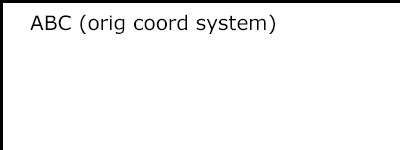Example NewCoordSys establishes a new user coordinate system by specifying transform="translate(50,50)" on the third 'g' element below. The new user coordinate system has its origin at location (50,50) in the original coordinate system. The result of this transformation is that the coordinate (30,30) in the new user coordinate system gets mapped to coordinate (80,80) in the original coordinate system (i.e., the coordinates have been translated by 50 units in X and 50 units in Y).

```<?xml version="1.0" standalone="no"?>
<!DOCTYPE svg PUBLIC "-//W3C//DTD SVG 1.1//EN"
"http://www.w3.org/Graphics/SVG/1.1/DTD/svg11.dtd">
<svg width="400px" height="150px" version="1.1"
xmlns="http://www.w3.org/2000/svg">
<desc>Example NewCoordSys - New user coordinate system</desc>
<g fill="none" stroke="black" stroke-width="3" >
<!-- Draw the axes of the original coordinate system -->
<line x1="0" y1="1.5" x2="400" y2="1.5" />
<line x1="1.5" y1="0" x2="1.5" y2="150" />
</g>
<g>
<text x="30" y="30" font-size="20" font-family="Verdana" >
ABC (orig coord system)
</text>
</g>
<!-- Establish a new coordinate system, which is
shifted (i.e., translated) from the initial coordinate
system by 50 user units along each axis. -->
<g transform="translate(50,50)">
<g fill="none" stroke="red" stroke-width="3" >
<!-- Draw lines of length 50 user units along
the axes of the new coordinate system -->
<line x1="0" y1="0" x2="50" y2="0" stroke="red" />
<line x1="0" y1="0" x2="0" y2="50" />
</g>
<text x="30" y="30" font-size="20" font-family="Verdana" >
ABC (translated coord system)
</text>
</g>
</svg>
```Example RotateScale illustrates simple rotate and scale transformations. The example defines two new coordinate systems:

• one which is the result of a translation by 50 units in X and 30 units in Y, followed by a rotation of 30 degrees
• another which is the result of a translation by 200 units in X and 40 units in Y, followed by a scale transformation of 1.5.
```<?xml version="1.0" standalone="no"?>
<!DOCTYPE svg PUBLIC "-//W3C//DTD SVG 1.1//EN"
"http://www.w3.org/Graphics/SVG/1.1/DTD/svg11.dtd">
<svg width="400px" height="120px" version="1.1"
xmlns="http://www.w3.org/2000/svg">
<desc>Example RotateScale - Rotate and scale transforms</desc>
<g fill="none" stroke="black" stroke-width="3" >
<!-- Draw the axes of the original coordinate system -->
<line x1="0" y1="1.5" x2="400" y2="1.5" />
<line x1="1.5" y1="0" x2="1.5" y2="120" />
</g>
<!-- Establish a new coordinate system whose origin is at (50,30)
in the initial coord. system and which is rotated by 30 degrees. -->
<g transform="translate(50,30)">
<g transform="rotate(30)">
<g fill="none" stroke="red" stroke-width="3" >
<line x1="0" y1="0" x2="50" y2="0" />
<line x1="0" y1="0" x2="0" y2="50" />
</g>
<text x="0" y="0" font-size="20" font-family="Verdana" fill="blue" >
ABC (rotate)
</text>
</g>
</g>
<!-- Establish a new coordinate system whose origin is at (200,40)
in the initial coord. system and which is scaled by 1.5. -->
<g transform="translate(200,40)">
<g transform="scale(1.5)">
<g fill="none" stroke="red" stroke-width="3" >
<line x1="0" y1="0" x2="50" y2="0" />
<line x1="0" y1="0" x2="0" y2="50" />
</g>
<text x="0" y="0" font-size="20" font-family="Verdana" fill="blue" >
ABC (scale)
</text>
</g>
</g>
</svg>
```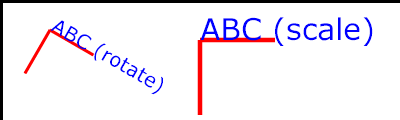Example Skew defines two coordinate systems which are skewed relative to the origin coordinate system.

```<?xml version="1.0" standalone="no"?>
<!DOCTYPE svg PUBLIC "-//W3C//DTD SVG 1.1//EN"
"http://www.w3.org/Graphics/SVG/1.1/DTD/svg11.dtd">
<svg width="400px" height="120px" version="1.1"
xmlns="http://www.w3.org/2000/svg">
<desc>Example Skew - Show effects of skewX and skewY</desc>
<g fill="none" stroke="black" stroke-width="3" >
<!-- Draw the axes of the original coordinate system -->
<line x1="0" y1="1.5" x2="400" y2="1.5" />
<line x1="1.5" y1="0" x2="1.5" y2="120" />
</g>
<!-- Establish a new coordinate system whose origin is at (30,30)
in the initial coord. system and which is skewed in X by 30 degrees. -->
<g transform="translate(30,30)">
<g transform="skewX(30)">
<g fill="none" stroke="red" stroke-width="3" >
<line x1="0" y1="0" x2="50" y2="0" />
<line x1="0" y1="0" x2="0" y2="50" />
</g>
<text x="0" y="0" font-size="20" font-family="Verdana" fill="blue" >
ABC (skewX)
</text>
</g>
</g>
<!-- Establish a new coordinate system whose origin is at (200,30)
in the initial coord. system and which is skewed in Y by 30 degrees. -->
<g transform="translate(200,30)">
<g transform="skewY(30)">
<g fill="none" stroke="red" stroke-width="3" >
<line x1="0" y1="0" x2="50" y2="0" />
<line x1="0" y1="0" x2="0" y2="50" />
</g>
<text x="0" y="0" font-size="20" font-family="Verdana" fill="blue" >
ABC (skewY)
</text>
</g>
</g>
</svg>
```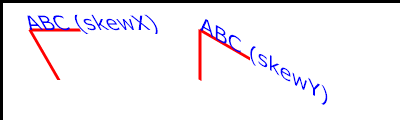Mathematically, all transformations can be represented as 3x3 transformation matrices of the following form: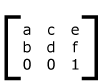Since only six values are used in the above 3x3 matrix, a transformation matrix is also expressed as a vector: [a b c d e f].

Transformations map coordinates and lengths from a new coordinate system into a previous coordinate system: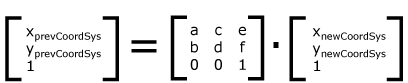Simple transformations are represented in matrix form as follows:

• Translation is equivalent to the matrix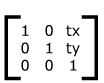or [1 0 0 1 tx ty], where tx and ty are the distances to translate coordinates in X and Y, respectively.

• Scaling is equivalent to the matrixor [sx 0 0 sy 0 0]. One unit in the X and Y directions in the new coordinate system equals sx and sy units in the previous coordinate system, respectively.

• Rotation about the origin is equivalent to the matrix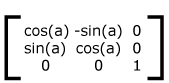or [cos(a) sin(a) -sin(a) cos(a) 0 0], which has the effect of rotating the coordinate system axes by angle a.

• A skew transformation along the x-axis is equivalent to the matrix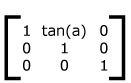or [1 0 tan(a) 1 0 0], which has the effect of skewing X coordinates by angle a.

• A skew transformation along the y-axis is equivalent to the matrix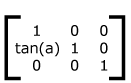or [1 tan(a) 0 1 0 0], which has the effect of skewing Y coordinates by angle a.

7.5 Nested transformations

Transformations can be nested to any level. The effect of nested transformations is to post-multiply (i.e., concatenate) the subsequent transformation matrices onto previously defined transformations: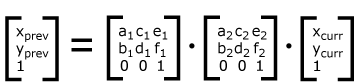For each given element, the accumulation of all transformations that have been defined on the given element and all of its ancestors up to and including the element that established the current viewport (usually, the 'svg' element which is the most immediate ancestor to the given element) is called the current transformation matrix or CTM. The CTM thus represents the mapping of current user coordinates to viewport coordinates:Example Nested illustrates nested transformations.

```<?xml version="1.0" standalone="no"?>
<!DOCTYPE svg PUBLIC "-//W3C//DTD SVG 1.1//EN"
"http://www.w3.org/Graphics/SVG/1.1/DTD/svg11.dtd">
<svg width="400px" height="150px" version="1.1"
xmlns="http://www.w3.org/2000/svg">
<desc>Example Nested - Nested transformations</desc>
<g fill="none" stroke="black" stroke-width="3" >
<!-- Draw the axes of the original coordinate system -->
<line x1="0" y1="1.5" x2="400" y2="1.5" />
<line x1="1.5" y1="0" x2="1.5" y2="150" />
</g>
<!-- First, a translate -->
<g transform="translate(50,90)">
<g fill="none" stroke="red" stroke-width="3" >
<line x1="0" y1="0" x2="50" y2="0" />
<line x1="0" y1="0" x2="0" y2="50" />
</g>
<text x="0" y="0" font-size="16" font-family="Verdana" >
....Translate(1)
</text>
<!-- Second, a rotate -->
<g transform="rotate(-45)">
<g fill="none" stroke="green" stroke-width="3" >
<line x1="0" y1="0" x2="50" y2="0" />
<line x1="0" y1="0" x2="0" y2="50" />
</g>
<text x="0" y="0" font-size="16" font-family="Verdana" >
....Rotate(2)
</text>
<!-- Third, another translate -->
<g transform="translate(130,160)">
<g fill="none" stroke="blue" stroke-width="3" >
<line x1="0" y1="0" x2="50" y2="0" />
<line x1="0" y1="0" x2="0" y2="50" />
</g>
<text x="0" y="0" font-size="16" font-family="Verdana" >
....Translate(3)
</text>
</g>
</g>
</g>
</svg>
```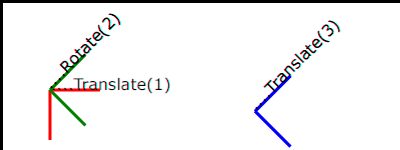In the example above, the CTM within the third nested transformation (i.e., the transform="translate(130,160)") consists of the concatenation of the three transformations, as follows:7.6 The transform attribute

The value of the transform attribute is a <transform-list>, which is defined as a list of transform definitions, which are applied in the order provided. The individual transform definitions are separated by whitespace and/or a comma. The available types of transform definitions include:

• matrix(<a> <b> <c> <d> <e> <f>), which specifies a transformation in the form of a transformation matrix of six values. matrix(a,b,c,d,e,f) is equivalent to applying the transformation matrix [a b c d e f].

• translate(<tx> [<ty>]), which specifies a translation by tx and ty. If <ty> is not provided, it is assumed to be zero.

• scale(<sx> [<sy>]), which specifies a scale operation by sx and sy. If <sy> is not provided, it is assumed to be equal to <sx>.

• rotate(<rotate-angle> [<cx> <cy>]), which specifies a rotation by <rotate-angle> degrees about a given point.
If optional parameters <cx> and <cy> are not supplied, the rotate is about the origin of the current user coordinate system. The operation corresponds to the matrix [cos(a) sin(a) -sin(a) cos(a) 0 0].
If optional parameters <cx> and <cy> are supplied, the rotate is about the point (<cx>, <cy>). The operation represents the equivalent of the following specification: translate(<cx>, <cy>) rotate(<rotate-angle>) translate(-<cx>, -<cy>).

• skewX(<skew-angle>), which specifies a skew transformation along the x-axis.

• skewY(<skew-angle>), which specifies a skew transformation along the y-axis.

All numeric values are real <number>s.

If a list of transforms is provided, then the net effect is as if each transform had been specified separately in the order provided. For example,

```<g transform="translate(-10,-20) scale(2) rotate(45) translate(5,10)">
<!-- graphics elements go here -->
</g>
```

is functionally equivalent to:

```<g transform="translate(-10,-20)">
<g transform="scale(2)">
<g transform="rotate(45)">
<g transform="translate(5,10)">
<!-- graphics elements go here -->
</g>
</g>
</g>
</g>
```

The transform attribute is applied to an element before processing any other coordinate or length values supplied for that element. In the element

```<rect x="10" y="10" width="20" height="20" transform="scale(2)"/>
```

the x, y, width and height values are processed after the current coordinate system has been scaled uniformly by a factor of 2 by the transform attribute. Attributes x, y, width and height (and any other attributes or properties) are treated as values in the new user coordinate system, not the previous user coordinate system. Thus, the above 'rect' element is functionally equivalent to:

```<g transform="scale(2)">
<rect x="10" y="10" width="20" height="20"/>
</g>
```

The following is the Backus-Naur Form (BNF) for values for the transform attribute. The following notation is used:

• *: 0 or more
• +: 1 or more
• ?: 0 or 1
• (): grouping
• |: separates alternatives
• double quotes surround literals
```transform-list:
wsp* transforms? wsp*
transforms:
transform
| transform comma-wsp+ transforms
transform:
matrix
| translate
| scale
| rotate
| skewX
| skewY
matrix:
"matrix" wsp* "(" wsp*
number comma-wsp
number comma-wsp
number comma-wsp
number comma-wsp
number comma-wsp
number wsp* ")"
translate:
"translate" wsp* "(" wsp* number ( comma-wsp number )? wsp* ")"
scale:
"scale" wsp* "(" wsp* number ( comma-wsp number )? wsp* ")"
rotate:
"rotate" wsp* "(" wsp* number ( comma-wsp number comma-wsp number )? wsp* ")"
skewX:
"skewX" wsp* "(" wsp* number wsp* ")"
skewY:
"skewY" wsp* "(" wsp* number wsp* ")"
number:
sign? integer-constant
| sign? floating-point-constant
comma-wsp:
(wsp+ comma? wsp*) | (comma wsp*)
comma:
","
integer-constant:
digit-sequence
floating-point-constant:
fractional-constant exponent?
| digit-sequence exponent
fractional-constant:
digit-sequence? "." digit-sequence
| digit-sequence "."
exponent:
( "e" | "E" ) sign? digit-sequence
sign:
"+" | "-"
digit-sequence:
digit
| digit digit-sequence
digit:
"0" | "1" | "2" | "3" | "4" | "5" | "6" | "7" | "8" | "9"
wsp:
(#x20 | #x9 | #xD | #xA)
```

For the transform attribute:

Animatable: yes.

See the 'animateTransform' element for information on animating transformations.

7.7 The viewBox attribute

It is often desirable to specify that a given set of graphics stretch to fit a particular container element. The viewBox attribute provides this capability.

All elements that establish a new viewport (see elements that establish viewports), plus the 'marker', 'pattern' and 'view' elements have attribute viewBox. The value of the viewBox attribute is a list of four numbers <min-x>, <min-y>, <width> and <height>, separated by whitespace and/or a comma, which specify a rectangle in user space which should be mapped to the bounds of the viewport established by the given element, taking into account attribute preserveAspectRatio. If specified, an additional transformation is applied to all descendants of the given element to achieve the specified effect.

A negative value for <width> or <height> is an error (see Error processing). A value of zero disables rendering of the element.

Example ViewBox illustrates the use of the viewBox attribute on the outermost 'svg' element to specify that the SVG content should stretch to fit bounds of the viewport.

```<?xml version="1.0" standalone="no"?>
<!DOCTYPE svg PUBLIC "-//W3C//DTD SVG 1.1//EN"
"http://www.w3.org/Graphics/SVG/1.1/DTD/svg11.dtd">
<svg width="300px" height="200px" version="1.1"
viewBox="0 0 1500 1000" preserveAspectRatio="none"
xmlns="http://www.w3.org/2000/svg">
<desc>Example ViewBox - uses the viewBox
attribute to automatically create an initial user coordinate
system which causes the graphic to scale to fit into the
viewport no matter what size the viewport is.</desc>
<!-- This rectangle goes from (0,0) to (1500,1000) in user space.
Because of the viewBox attribute above,
the rectangle will end up filling the entire area
reserved for the SVG content. -->
<rect x="0" y="0" width="1500" height="1000"
fill="yellow" stroke="blue" stroke-width="12"  />
<!-- A large, red triangle -->
<path fill="red"  d="M 750,100 L 250,900 L 1250,900 z"/>
<!-- A text string that spans most of the viewport -->
<text x="100" y="600" font-size="200" font-family="Verdana" >
Stretch to fit
</text>
</svg>
```
Example ViewBox
Rendered into
viewport with
width=300px,
height=200px
Rendered into
viewport with
width=150px,
height=200px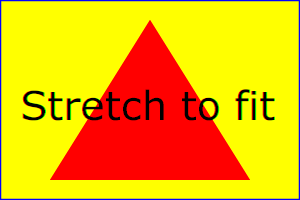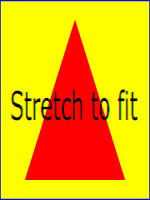The effect of the viewBox attribute is that the user agent automatically supplies the appropriate transformation matrix to map the specified rectangle in user space to the bounds of a designated region (often, the viewport). To achieve the effect of the example on the left, with viewport dimensions of 300 by 200 pixels, the user agent needs to automatically insert a transformation which scales both X and Y by 0.2. The effect is equivalent to having a viewport of size 300px by 200px and the following supplemental transformation in the document, as follows:

```<?xml version="1.0" standalone="no"?>
<!DOCTYPE svg PUBLIC "-//W3C//DTD SVG 1.1//EN"
"http://www.w3.org/Graphics/SVG/1.1/DTD/svg11.dtd">
<svg width="300px" height="200px" version="1.1"
xmlns="http://www.w3.org/2000/svg">
<g transform="scale(0.2)">
<!-- Rest of document goes here -->
</g>
</svg>
```
To achieve the effect of the example on the right, with viewport dimensions of 150 by 200 pixels, the user agent needs to automatically insert a transformation which scales X by 0.1 and Y by 0.2. The effect is equivalent to having a viewport of size 150px by 200px and the following supplemental transformation in the document, as follows:
```<?xml version="1.0" standalone="no"?>
<!DOCTYPE svg PUBLIC "-//W3C//DTD SVG 1.1//EN"
"http://www.w3.org/Graphics/SVG/1.1/DTD/svg11.dtd">
<svg width="150px" height="200px" version="1.1"
xmlns="http://www.w3.org/2000/svg">
<g transform="scale(0.1 0.2)">
<!-- Rest of document goes here -->
</g>
</svg>
```

(Note: in some cases the user agent will need to supply a translate transformation in addition to a scale transformation. For example, on an outermost 'svg', a translate transformation will be needed if the viewBox attributes specifies values other than zero for <min-x> or <min-y>.)

Unlike the transform attribute (see effect of the transform on sibling attributes), the automatic transformation that is created due to a viewBox does not affect the x, y, width and height attributes (or in the case of the 'marker' element, the markerWidth and markerHeight attributes) on the element with the viewBox attribute. Thus, in the example above which shows an 'svg' element which has attributes width, height and viewBox, the width and height attributes represent values in the coordinate system that exists before the viewBox transformation is applied. On the other hand, like the transform attribute, it does establish a new coordinate system for all other attributes and for descendant elements.

For the viewBox attribute:

Animatable: yes.

7.8 The preserveAspectRatio attribute

In some cases, typically when using the viewBox attribute, it is desirable that the graphics stretch to fit non-uniformly to take up the entire viewport. In other cases, it is desirable that uniform scaling be used for the purposes of preserving the aspect ratio of the graphics.

Attribute preserveAspectRatio="[defer] <align> [<meetOrSlice>]", which is available for all elements that establish a new viewport (see elements that establish viewports), plus the 'image', 'marker', 'pattern' and 'view' elements, indicates whether or not to force uniform scaling.

For elements that establish a new viewport (see elements that establish viewports), plus the 'marker', 'pattern' and 'view' elements, preserveAspectRatio only applies when a value has been provided for viewBox on the same element. For these elements, if attribute viewBox is not provided, then preserveAspectRatio is ignored.

For 'image' elements, preserveAspectRatio indicates how referenced images should be fitted with respect to the reference rectangle and whether the aspect ratio of the referenced image should be preserved with respect to the current user coordinate system.

If the value of preserveAspectRatio on an 'image' element starts with 'defer' then the value of the preserveAspectRatio attribute on the referenced content if present should be used.  If the referenced content lacks a value for preserveAspectRatio then the preserveAspectRatio attribute should be processed as normal (ignoring 'defer').  For preserveAspectRatio on all other elements the 'defer' portion of the attribute is ignored.

The <align> parameter indicates whether to force uniform scaling and, if so, the alignment method to use in case the aspect ratio of the viewBox doesn't match the aspect ratio of the viewport. The <align> parameter must be one of the following strings:

• none - Do not force uniform scaling. Scale the graphic content of the given element non-uniformly if necessary such that the element's bounding box exactly matches the viewport rectangle.
(Note: if <align> is none, then the optional <meetOrSlice> value is ignored.)
• xMinYMin - Force uniform scaling.
Align the <min-x> of the element's viewBox with the smallest X value of the viewport.
Align the <min-y> of the element's viewBox with the smallest Y value of the viewport.
• xMidYMin - Force uniform scaling.
Align the midpoint X value of the element's viewBox with the midpoint X value of the viewport.
Align the <min-y> of the element's viewBox with the smallest Y value of the viewport.
• xMaxYMin - Force uniform scaling.
Align the <min-x>+<width> of the element's viewBox with the maximum X value of the viewport.
Align the <min-y> of the element's viewBox with the smallest Y value of the viewport.
• xMinYMid - Force uniform scaling.
Align the <min-x> of the element's viewBox with the smallest X value of the viewport.
Align the midpoint Y value of the element's viewBox with the midpoint Y value of the viewport.
• xMidYMid (the default) - Force uniform scaling.
Align the midpoint X value of the element's viewBox with the midpoint X value of the viewport.
Align the midpoint Y value of the element's viewBox with the midpoint Y value of the viewport.
• xMaxYMid - Force uniform scaling.
Align the <min-x>+<width> of the element's viewBox with the maximum X value of the viewport.
Align the midpoint Y value of the element's viewBox with the midpoint Y value of the viewport.
• xMinYMax - Force uniform scaling.
Align the <min-x> of the element's viewBox with the smallest X value of the viewport.
Align the <min-y>+<height> of the element's viewBox with the maximum Y value of the viewport.
• xMidYMax - Force uniform scaling.
Align the midpoint X value of the element's viewBox with the midpoint X value of the viewport.
Align the <min-y>+<height> of the element's viewBox with the maximum Y value of the viewport.
• xMaxYMax - Force uniform scaling.
Align the <min-x>+<width> of the element's viewBox with the maximum X value of the viewport.
Align the <min-y>+<height> of the element's viewBox with the maximum Y value of the viewport.

The <meetOrSlice> parameter is optional and, if provided, is separated from the <align> value by one or more spaces and then must be one of the following strings:

• meet (the default) - Scale the graphic such that:
• aspect ratio is preserved
• the entire viewBox is visible within the viewport
• the viewBox is scaled up as much as possible, while still meeting the other criteria
In this case, if the aspect ratio of the graphic does not match the viewport, some of the viewport will extend beyond the bounds of the viewBox (i.e., the area into which the viewBox will draw will be smaller than the viewport).
• slice - Scale the graphic such that:
• aspect ratio is preserved
• the entire viewport is covered by the viewBox
• the viewBox is scaled down as much as possible, while still meeting the other criteria
In this case, if the aspect ratio of the viewBox does not match the viewport, some of the viewBox will extend beyond the bounds of the viewport (i.e., the area into which the viewBox will draw is larger than the viewport).

Example PreserveAspectRatio illustrates the various options on preserveAspectRatio. To save space, XML entities have been defined for the three repeated graphic objects, the rectangle with the smile inside and the outlines of the two rectangles which have the same dimensions as the target viewports. The example creates several new viewports by including 'svg' sub-elements embedded inside the outermost 'svg' element (see Establishing a new viewport).

```<?xml version="1.0" encoding="UTF-8"?>
<!DOCTYPE svg PUBLIC "-//W3C//DTD SVG 1.1//EN"
"http://www.w3.org/Graphics/SVG/1.1/DTD/svg11.dtd"
[ <!ENTITY Smile "
<rect x='.5' y='.5' width='29' height='39' fill='black' stroke='red'/>
<g transform='translate(0, 5)'>
<circle cx='15' cy='15' r='10' fill='yellow'/>
<circle cx='12' cy='12' r='1.5' fill='black'/>
<circle cx='17' cy='12' r='1.5' fill='black'/>
<path d='M 10 19 A 8 8 0 0 0 20 19' stroke='black' stroke-width='2'/>
</g>
">
<!ENTITY Viewport1 "<rect x='.5' y='.5' width='49' height='29'
fill='none' stroke='blue'/>">
<!ENTITY Viewport2 "<rect x='.5' y='.5' width='29' height='59'
fill='none' stroke='blue'/>">
]>
<svg width="450px" height="300px" version="1.1"
xmlns="http://www.w3.org/2000/svg">
<desc>Example PreserveAspectRatio - illustrates preserveAspectRatio attribute</desc>
<rect x="1" y="1" width="448" height="298"
fill="none" stroke="blue"/>
<g font-size="9">
<text x="10" y="30">SVG to fit</text>
<g transform="translate(20,40)">&Smile;</g>
<text x="10" y="110">Viewport 1</text>
<g transform="translate(10,120)">&Viewport1;</g>
<text x="10" y="180">Viewport 2</text>
<g transform="translate(20,190)">&Viewport2;</g>
<g id="meet-group-1" transform="translate(100, 60)">
<text x="0" y="-30">--------------- meet ---------------</text>
<g><text y="-10">xMin*</text>&Viewport1;
<svg preserveAspectRatio="xMinYMin meet" viewBox="0 0 30 40"
width="50" height="30">&Smile;</svg></g>
<g transform="translate(70,0)"><text y="-10">xMid*</text>&Viewport1;
<svg preserveAspectRatio="xMidYMid meet" viewBox="0 0 30 40"
width="50" height="30">&Smile;</svg></g>
<g transform="translate(0,70)"><text y="-10">xMax*</text>&Viewport1;
<svg preserveAspectRatio="xMaxYMax meet" viewBox="0 0 30 40"
width="50" height="30">&Smile;</svg></g>
</g>
<g id="meet-group-2" transform="translate(250, 60)">
<text x="0" y="-30">---------- meet ----------</text>
<g><text y="-10">*YMin</text>&Viewport2;
<svg preserveAspectRatio="xMinYMin meet" viewBox="0 0 30 40"
width="30" height="60">&Smile;</svg></g>
<g transform="translate(50, 0)"><text y="-10">*YMid</text>&Viewport2;
<svg preserveAspectRatio="xMidYMid meet" viewBox="0 0 30 40"
width="30" height="60">&Smile;</svg></g>
<g transform="translate(100, 0)"><text y="-10">*YMax</text>&Viewport2;
<svg preserveAspectRatio="xMaxYMax meet" viewBox="0 0 30 40"
width="30" height="60">&Smile;</svg></g>
</g>
<g id="slice-group-1" transform="translate(100, 220)">
<text x="0" y="-30">---------- slice ----------</text>
<g><text y="-10">xMin*</text>&Viewport2;
<svg preserveAspectRatio="xMinYMin slice" viewBox="0 0 30 40"
width="30" height="60">&Smile;</svg></g>
<g transform="translate(50,0)"><text y="-10">xMid*</text>&Viewport2;
<svg preserveAspectRatio="xMidYMid slice" viewBox="0 0 30 40"
width="30" height="60">&Smile;</svg></g>
<g transform="translate(100,0)"><text y="-10">xMax*</text>&Viewport2;
<svg preserveAspectRatio="xMaxYMax slice" viewBox="0 0 30 40"
width="30" height="60">&Smile;</svg></g>
</g>
<g id="slice-group-2" transform="translate(250, 220)">
<text x="0" y="-30">--------------- slice ---------------</text>
<g><text y="-10">*YMin</text>&Viewport1;
<svg preserveAspectRatio="xMinYMin slice" viewBox="0 0 30 40"
width="50" height="30">&Smile;</svg></g>
<g transform="translate(70,0)"><text y="-10">*YMid</text>&Viewport1;
<svg preserveAspectRatio="xMidYMid slice" viewBox="0 0 30 40"
width="50" height="30">&Smile;</svg></g>
<g transform="translate(140,0)"><text y="-10">*YMax</text>&Viewport1;
<svg preserveAspectRatio="xMaxYMax slice" viewBox="0 0 30 40"
width="50" height="30">&Smile;</svg></g>
</g>
</g>
</svg>
```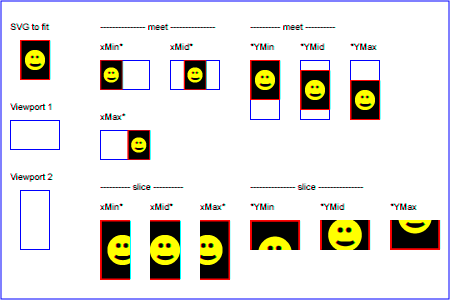For the preserveAspectRatio attribute:

Animatable: yes.

7.9 Establishing a new viewport

At any point in an SVG drawing, you can establish a new viewport into which all contained graphics is drawn by including an 'svg' element inside SVG content. By establishing a new viewport, you also implicitly establish a new viewport coordinate system, a new user coordinate system, and, potentially, a new clipping path (see the definition of the 'overflow' property). Additionally, there is a new meaning for percentage units defined to be relative to the current viewport since a new viewport has been established (see Units)

The bounds of the new viewport are defined by the x, y, width and height attributes on the element establishing the new viewport, such as an 'svg' element. Both the new viewport coordinate system and the new user coordinate system have their origins at (x, y), where x and y represent the value of the corresponding attributes on the element establishing the viewport. The orientation of the new viewport coordinate system and the new user coordinate system correspond to the orientation of the current user coordinate system for the element establishing the viewport. A single unit in the new viewport coordinate system and the new user coordinate system are the same size as a single unit in the current user coordinate system for the element establishing the viewport.

Here is an example:

```<?xml version="1.0" standalone="no"?>
<!DOCTYPE svg PUBLIC "-//W3C//DTD SVG 1.1//EN"
"http://www.w3.org/Graphics/SVG/1.1/DTD/svg11.dtd">
<svg width="4in" height="3in" version="1.1"
xmlns="http://www.w3.org/2000/svg">
<desc>This SVG drawing embeds another one,
thus establishing a new viewport
</desc>
<!-- The following statement establishing a new viewport
and renders SVG drawing B into that viewport -->
<svg x="25%" y="25%" width="50%" height="50%">
<!-- drawing B goes here -->
</svg>
</svg>
```

For an extensive example of creating new viewports, see Example PreserveAspectRatio.

The following elements establish new viewports:

• The 'svg' element
• A 'symbol' element define new viewports whenever they are instanced by a 'use' element.
• An 'image' element that references an SVG file will result in the establishment of a temporary new viewport since the referenced resource by definition will have an 'svg' element.
• A 'foreignObject' element creates a new viewport for rendering the content that is within the element.

Whether a new viewport also establishes a new additional clipping path is determined by the value of the 'overflow' property on the element that establishes the new viewport. If a clipping path is created to correspond to the new viewport, the clipping path's geometry is determined by the value of the 'clip' property. Also, see Clip to viewport vs. clip to viewBox.

7.10 Units

All coordinates and lengths in SVG can be specified with or without a unit identifier.

When a coordinate or length value is a number without a unit identifier (e.g., "25"), then the given coordinate or length is assumed to be in user units (i.e., a value in the current user coordinate system). For example:

```<text style="font-size: 50">Text size is 50 user units</text>
```

Alternatively, a coordinate or length value can be expressed as a number following by a unit identifier (e.g., "25cm" or "15em"). The list of unit identifiers in SVG matches the list of unit identifiers in CSS: em, ex, px, pt, pc, cm, mm, in and percentages. The following describes how the various unit identifiers are processed:

• As in CSS, the em and ex unit identifiers are relative to the current font's font-size and x-height, respectively.

• One px unit is defined to be equal to one user unit. Thus, a length of "5px" is the same as a length of "5".

Note that at initialization, a user unit in the the initial coordinate system is equivalenced to the parent environment's notion of a px unit. Thus, in the the initial coordinate system, because the user coordinate system aligns exactly with the parent's coordinate system, and because often the parent's coordinate system aligns with the device pixel grid, "5px" might actually map to 5 devices pixels. However, if there are any coordinate system transformation due to the use of transform or viewBox attributes, because "5px" maps to 5 user units and because the coordinate system transformations have resulted in a revised user coordinate system, "5px" likely will not map to 5 device pixels. As a result, in most circumstances, "px" units will not map to the device pixel grid.

• The other absolute unit identifiers from CSS (i.e., pt, pc, cm, mm, in) are all defined as an appropriate multiple of one px unit (which, according to the previous item, is defined to be equal to one user unit), based on what the SVG user agent determines is the size of a px unit (possibly passed from the parent processor or environment at initialization time). For example, suppose that the user agent can determine from its environment that "1px" corresponds to "0.2822222mm" (i.e., 90dpi). Then, for all processing of SVG content:

• "1pt" equals "1.25px" (and therefore 1.25 user units)
• "1pc" equals "15px" (and therefore 15 user units)
• "1mm" would be "3.543307px" (3.543307 user units)
• "1cm" equals "35.43307px" (and therefore 35.43307 user units)
• "1in" equals "90px" (and therefore 90 user units)

Note that use of px units or any other absolute unit identifiers can cause inconsistent visual results on different viewing environments since the size of "1px" may map to a different number of user units on different systems; thus, absolute units identifiers are only recommended for the width and the height on outermost 'svg' elements and situations where the content contains no transformations and it is desirable to specify values relative to the device pixel grid or to a particular real world unit size.

For percentage values that are defined to be relative to the size of viewport:

• For any x-coordinate value or width value expressed as a percentage of the viewport, the value to use is the specified percentage of the actual-width in user units for the nearest containing viewport, where actual-width is the width dimension of the viewport element within the user coordinate system for the viewport element.
• For any y-coordinate value or height value expressed as a percentage of the viewport, the value to use is the specified percentage of the actual-height in user units for the nearest containing viewport, where actual-height is the height dimension of the viewport element within the user coordinate system for the viewport element.
• For any other length value expressed as a percentage of the viewport, the percentage is calculated as the specified percentage of ```sqrt((actual-width)**2 + (actual-height)**2))/sqrt(2)```.

Example Units below illustrates some of the processing rules for different types of units.

```<?xml version="1.0" standalone="no"?>
<!DOCTYPE svg PUBLIC "-//W3C//DTD SVG 1.1//EN"
"http://www.w3.org/Graphics/SVG/1.1/DTD/svg11.dtd">
<svg width="400px" height="200px" viewBox="0 0 4000 2000"
xmlns="http://www.w3.org/2000/svg" version="1.1">
<title>Example Units</title>
<desc>Illustrates various units options</desc>
<!-- Frame the picture -->
<rect x="5" y="5" width="3990" height="1990"
fill="none" stroke="blue" stroke-width="10"/>
<g fill="blue" stroke="red" font-family="Verdana" font-size="150">
<!-- Absolute unit specifiers -->
<g transform="translate(400,0)">
<text x="-50" y="300" fill="black" stroke="none">Abs. units:</text>
<rect x="0" y="400" width="4in" height="2in" stroke-width=".4in"/>
<rect x="0" y="750" width="384" height="192" stroke-width="38.4"/>
<g transform="scale(2)">
<rect x="0" y="600" width="4in" height="2in" stroke-width=".4in"/>
</g>
</g>
<!-- Relative unit specifiers -->
<g transform="translate(1600,0)">
<text x="-50" y="300" fill="black" stroke="none">Rel. units:</text>
<rect x="0" y="400" width="2.5em" height="1.25em" stroke-width=".25em"/>
<rect x="0" y="750" width="375" height="187.5" stroke-width="37.5"/>
<g transform="scale(2)">
<rect x="0" y="600" width="2.5em" height="1.25em" stroke-width=".25em"/>
</g>
</g>
<!-- Percentages -->
<g transform="translate(2800,0)">
<text x="-50" y="300" fill="black" stroke="none">Percentages:</text>
<rect x="0" y="400" width="10%" height="10%" stroke-width="1%"/>
<rect x="0" y="750" width="400" height="200" stroke-width="31.62"/>
<g transform="scale(2)">
<rect x="0" y="600" width="10%" height="10%" stroke-width="1%"/>
</g>
</g>
</g>
</svg>
```The three rectangles on the left demonstrate the use of one of the absolute unit identifiers, the "in" unit (inch). The reference image above was generated on a 96dpi system (i.e., 1 inch = 96 pixels). Therefore, the topmost rectangle, which is specified in inches, is exactly the same size as the middle rectangle, which is specified in user units such that there are 96 user units for each corresponding inch in the topmost rectangle. (Note: on systems with different screen resolutions, the top and middle rectangles will likely be rendered at different sizes.) The bottom rectangle of the group illustrates what happens when values specified in inches are scaled.

The three rectangles in the middle demonstrate the use of one of the relative unit identifiers, the "em" unit. Because the 'font-size' property has been set to 150 on the outermost 'g' element, each "em" unit is equal to 150 user units. The topmost rectangle, which is specified in "em" units, is exactly the same size as the middle rectangle, which is specified in user units such that there are 150 user units for each corresponding "em" unit in the topmost rectangle. The bottom rectangle of the group illustrates what happens when values specified in "em" units are scaled.

The three rectangles on the right demonstrate the use of percentages. Note that the width and height of the viewport in the user coordinate system for the viewport element (in this case, the outermost 'svg' element) are 4000 and 2000, respectively, because processing the viewBox attribute results in a transformed user coordinate system. The topmost rectangle, which is specified in percentage units, is exactly the same size as the middle rectangle, which is specified in equivalent user units. In particular, note that the 'stroke-width' property in the middle rectangle is set to 1% of the ```sqrt((actual-width)**2 + (actual-height)**2)) / sqrt(2)```, which in this case is .01*sqrt(4000*4000+2000*2000)/sqrt(2), or 31.62. The bottom rectangle of the group illustrates what happens when values specified in percentage units are scaled.

7.11 Object bounding box units

The following elements offer the option of expressing coordinate values and lengths as fractions (and, in some cases, percentages) of the bounding box (via keyword objectBoundingBox) on a given element:

Element Attribute Effect
'linearGradient' gradientUnits="objectBoundingBox" Indicates that the attributes which specify the gradient vector (x1, y1, x2, y2) represent fractions or percentages of the bounding box of the element to which the gradient is applied.
'radialGradient' gradientUnits="objectBoundingBox" Indicates that the attributes which specify the center (cx, cy), the radius (r) and focus (fx, fy) represent fractions or percentages of the bounding box of the element to which the gradient is applied.
'pattern' patternUnits="objectBoundingBox" Indicates that the attributes which define how to tile the pattern (x, y, width, height) are established using the bounding box of the element to which the pattern is applied.
'pattern' patternContentUnits="objectBoundingBox" Indicates that the user coordinate system for the contents of the pattern is established using the bounding box of the element to which the pattern is applied.
'clipPath' clipPathUnits="objectBoundingBox" Indicates that the user coordinate system for the contents of the 'clipPath' element is established using the bounding box of the element to which the clipping path is applied.
'mask' maskUnits="objectBoundingBox" Indicates that the attributes which define the masking region (x, y, width, height) is established using the bounding box of the element to which the mask is applied.
'mask' maskContentUnits="objectBoundingBox" Indicates that the user coordinate system for the contents of the 'mask' element are established using the bounding box of the element to which the mask is applied.
'filter' filterUnits="objectBoundingBox" Indicates that the attributes which define the filter effects region (x, y, width, height) represent fractions or percentages of the bounding box of the element to which the filter is applied.
'filter' primitiveUnits="objectBoundingBox" Indicates that the various length values within the filter primitives represent fractions or percentages of the bounding box of the element to which the filter is applied.

In the discussion that follows, the term applicable element is the element to which the given effect applies. For gradients and patterns, the applicable element is the graphics element which has its 'fill' or 'stroke' property referencing the given gradient or pattern. (See Inheritance of Painting Properties. For special rules concerning text elements, see the discussion of object bounding box units and text elements.) For clipping paths, masks and filters, the applicable element can be either a container element or a graphics element.

When keyword objectBoundingBox is used, then the effect is as if a supplemental transformation matrix were inserted into the list of nested transformation matrices to create a new user coordinate system.

First, the (minx,miny) and (maxx,maxy) coordinates are determined for the applicable element and all of its descendants. The values minx, miny, maxx and maxy are determined by computing the maximum extent of the shape of the element in X and Y with respect to the user coordinate system for the applicable element. The bounding box is the tightest fitting rectangle aligned with the axes of the applicable element's user coordinate system that entirely encloses the applicable element and its descendants. The bounding box is computed exclusive of any values for clipping, masking, filter effects, opacity and stroke-width. For curved shapes, the bounding box encloses all portions of the shape, not just end points. For 'text' elements, for the purposes of the bounding box calculation, each glyph is treated as a separate graphics element. The calculations assume that all glyphs occupy the full glyph cell. For example, for horizontal text, the calculations assume that each glyph extends vertically to the full ascent and descent values for the font.

Then, coordinate (0,0) in the new user coordinate system is mapped to the (minx,miny) corner of the tight bounding box within the user coordinate system of the applicable element and coordinate (1,1) in the new user coordinate system is mapped to the (maxx,maxy) corner of the tight bounding box of the applicable element. In most situations, the following transformation matrix produces the correct effect:

```[ (maxx-minx) 0 0 (maxy-miny) minx miny ]
```

When percentages are used with attributes that define the gradient vector, the pattern tile, the filter region or the masking region, a percentage represents the same value as the corresponding decimal value (e.g., 50% means the same as 0.5). If percentages are used within the content of a 'pattern', 'clipPath', 'mask' or 'filter' element, these values are treated according to the processing rules for percentages as defined in Units.

Any numeric value can be specified for values expressed as a fraction or percentage of object bounding box units. In particular, fractions less are zero or greater than one and percentages less than 0% or greater than 100% can be specified.

Keyword objectBoundingBox should not be used when the geometry of the applicable element has no width or no height, such as the case of a horizontal or vertical line, even when the line has actual thickness when viewed due to having a non-zero stroke width since stroke width is ignored for bounding box calculations. When the geometry of the applicable element has no width or height and objectBoundingBox is specified, then the given effect (e.g., a gradient or a filter) will be ignored.

7.12 Geographic Coordinate Systems

In order to allow interoperability between SVG content generators and user agents dealing with maps encoded in SVG, SVG encourages the use of a common metadata definition for describing the coordinate system used to generate SVG documents.

Such metadata should be added under the 'metadata' element of the topmost 'svg' element describing the map. They consist of an RDF description of the Coordinate Reference System definition used to generate the SVG map.

The definition should be conformant to the XML grammar described in the OpenGIS Recommendation on the Definition of Coordinate Reference System [OpenGIS Coordinate Systems]. In order to correctly map the 2-dimensional data used by SVG, the CRS must be of subtype ProjectedCRS or Geographic2dCRS. The first axis of the described CRS maps the SVG x-axis and the second axis maps the SVG y-axis. Optionally, an additional affine transformation may have been applied during this mapping. This additional transformation is described by an SVG transform attribute that can be added to the OpenGIS 'CoordinateReferenceSystem' element. Note that the transform attribute on the 'CoordinateReferenceSystem' does not indicate that a transformation should be applied to the data within the file, it simply describes the transformation that was applied to the data when being encoded in SVG.

There are three typical uses for the SVG transform attribute. These are described below and used in the examples.

• Most ProjectedCRS have the north direction represented by positive values of the second axis and conversely SVG has a y-down coordinate system. That's why, in order to follow the usual way to represent a map with the north at its top, it is recommended for that kind of ProjectedCRS to use the SVG transform attribute with a 'scale(1, -1)' value as in the third example below.

• Most Geographic2dCRS have the latitude as their first axis rather than the longitude, which means that the south-north axis would be represented by the x-axis in SVG instead of the usual y-axis. That's why, in order to follow the usual way to represent a map with the north at its top, it is recommended for that kind of Geographic2dCRS to use the SVG transform attribute with a 'rotate(-90)' value as in the first example (while also adding the scale(1, -1) as for ProjectedCRS).

• In addition, when converting for profiles which place restrictions on precision of real number values, it may be useful to add an additional scaling factor to retain good precision for a specific area. When generating an SVG document from WGS84 geographic coordinates (EPGS 4326), we recommend the use of an additional 100 times scaling factor corresponding to an SVG transform attribute with a 'rotate(-90) scale(100)' value (shown in the second example). Different scaling values may be required depending on the particular CRS.

The main purpose of such metadata is to indicate to the User Agent that two or more SVG documents can be overlayed or merged into a single document. Obviously, if two maps reference the same Coordinate Reference System definition and have the same SVG transform attribute value then they can be overlayed without reprojecting the data. If the maps reference different Coordinate Reference Systems and/or have different SVG transform attribute values, then a specialized cartographic User Agent may choose to transform the coordinate data to overlay the data. However, typical SVG user agents are not required to perform these types of transformations, or even recognize the metadata.

Below is a simple example of the coordinate metadata, which describes the coordinate system used by the document via a URI.

```
<?xml version="1.0"?>
<!DOCTYPE svg PUBLIC "-//W3C//DTD SVG 1.1//EN"
"http://www.w3.org/Graphics/SVG/1.1/DTD/svg11.dtd">
<svg width="100" height="100" viewBox="0 0 1000 1000" version="1.1"
xmlns="http://www.w3.org/2000/svg">
<rdf:RDF xmlns:rdf = "http://www.w3.org/1999/02/22-rdf-syntax-ns#"
xmlns:crs = "http://www.ogc.org/crs"
xmlns:svg="http://wwww.w3.org/2000/svg">
<rdf:Description>
<!-- The Coordinate Reference System is described
through an URI. -->
<crs:CoordinateReferenceSystem svg:transform="rotate(-90)"
rdf:resource=""http://www.example.org/srs/epsg.xml#4326"/>
</rdf:Description>
</rdf:RDF>
<!-- The actual map content -->
</svg>
```

The second example uses a well-known identifier to describe the coordinate system. Note that the coordinates used in the document have had the supplied transform applied.

```<?xml version="1.0"?>
<!DOCTYPE svg PUBLIC "-//W3C//DTD SVG 1.1//EN"
"http://www.w3.org/Graphics/SVG/1.1/DTD/svg11.dtd">
<svg width="100" height="100" viewBox="0 0 1000 1000" version="1.1"
xmlns="http://www.w3.org/2000/svg">
<rdf:RDF xmlns:rdf = "http://www.w3.org/1999/02/22-rdf-syntax-ns#"
xmlns:crs = "http://www.ogc.org/crs"
xmlns:svg="http://wwww.w3.org/2000/svg">
<rdf:Description>
<!-- In case of a well-known Coordinate Reference System
an 'Identifier' is enough to describe the CRS -->
<crs:CoordinateReferenceSystem svg:transform="rotate(-90) scale(100, 100)">
<crs:Identifier>
<crs:code>4326</crs:code>
<crs:codeSpace>EPSG</crs:codeSpace>
<crs:edition>5.2</crs:edition>
</crs:Identifier>
</crs:CoordinateReferenceSystem>
</rdf:Description>
</rdf:RDF>
<!-- The actual map content -->
</svg>
```

The third example defines the coordinate system completely within the SVG document.

```<?xml version="1.0"?>
<!DOCTYPE svg PUBLIC "-//W3C//DTD SVG 1.1//EN"
"http://www.w3.org/Graphics/SVG/1.1/DTD/svg11.dtd">
<svg width="100" height="100" viewBox="0 0 1000 1000" version="1.1"
xmlns="http://www.w3.org/2000/svg">
<rdf:RDF xmlns:rdf="http://www.w3.org/1999/02/22-rdf-syntax-ns#"
xmlns:crs="http://www.ogc.org/crs"
xmlns:svg="http://wwww.w3.org/2000/svg">
<rdf:Description>
<!-- For other CRS it should be entirely defined -->
<crs:CoordinateReferenceSystem svg:transform="scale(1,-1)">
<crs:NameSet>
<crs:name>Mercator projection of WGS84</crs:name>
</crs:NameSet>
<crs:ProjectedCRS>
<!-- The actual definition of the CRS -->
<crs:CartesianCoordinateSystem>
<crs:dimension>2</crs:dimension>
<crs:CoordinateAxis>
<crs:axisDirection>north</crs:axisDirection>
<crs:AngularUnit>
<crs:Identifier>
<crs:code>9108</crs:code>
<crs:codeSpace>EPSG</crs:codeSpace>
<crs:edition>5.2</crs:edition>
</crs:Identifier>
</crs:AngularUnit>
</crs:CoordinateAxis>
<crs:CoordinateAxis>
<crs:axisDirection>east</crs:axisDirection>
<crs:AngularUnit>
<crs:Identifier>
<crs:code>9108</crs:code>
<crs:codeSpace>EPSG</crs:codeSpace>
<crs:edition>5.2</crs:edition>
</crs:Identifier>
</crs:AngularUnit>
</crs:CoordinateAxis>
</crs:CartesianCoordinateSystem>
<crs:CoordinateReferenceSystem>
<!-- the reference system of that projected system is
WGS84 which is EPSG 4326 in EPSG codeSpace -->
<crs:NameSet>
<crs:name>WGS 84</crs:name>
</crs:NameSet>
<crs:Identifier>
<crs:code>4326</crs:code>
<crs:codeSpace>EPSG</crs:codeSpace>
<crs:edition>5.2</crs:edition>
</crs:Identifier>
</crs:CoordinateReferenceSystem>
<crs:CoordinateTransformationDefinition>
<crs:sourceDimensions>2</crs:sourceDimensions>
<crs:targetDimensions>2</crs:targetDimensions>
<crs:ParameterizedTransformation>
<crs:TransformationMethod>
<!-- the projection is a Mercator projection which is
EPSG 9805 in EPSG codeSpace -->
<crs:NameSet>
<crs:name>Mercator</crs:name>
</crs:NameSet>
<crs:Identifier>
<crs:code>9805</crs:code>
<crs:codeSpace>EPSG</crs:codeSpace>
<crs:edition>5.2</crs:edition>
</crs:Identifier>
<crs:description>Mercator (2SP)</crs:description>
</crs:TransformationMethod>
<crs:Parameter>
<crs:NameSet>
<crs:name>Latitude of 1st standart parallel</crs:name>
</crs:NameSet>
<crs:Identifier>
<crs:code>8823</crs:code>
<crs:codeSpace>EPSG</crs:codeSpace>
<crs:edition>5.2</crs:edition>
</crs:Identifier>
<crs:value>0</crs:value>
</crs:Parameter>
<crs:Parameter>
<crs:NameSet>
<crs:name>Longitude of natural origin</crs:name>
</crs:NameSet>
<crs:Identifier>
<crs:code>8802</crs:code>
<crs:codeSpace>EPSG</crs:codeSpace>
<crs:edition>5.2</crs:edition>
</crs:Identifier>
<crs:value>0</crs:value>
</crs:Parameter>
<crs:Parameter>
<crs:NameSet>
<crs:name>False Easting</crs:name>
</crs:NameSet>
<crs:Identifier>
<crs:code>8806</crs:code>
<crs:codeSpace>EPSG</crs:codeSpace>
<crs:edition>5.2</crs:edition>
</crs:Identifier>
<crs:value>0</crs:value>
</crs:Parameter>
<crs:Parameter>
<crs:NameSet>
<crs:name>False Northing</crs:name>
</crs:NameSet>
<crs:Identifier>
<crs:code>8807</crs:code>
<crs:codeSpace>EPSG</crs:codeSpace>
<crs:edition>5.2</crs:edition>
</crs:Identifier>
<crs:value>0</crs:value>
</crs:Parameter>
</crs:ParameterizedTransformation>
</crs:CoordinateTransformationDefinition>
</crs:ProjectedCRS>
</crs:CoordinateReferenceSystem>
</rdf:Description>
</rdf:RDF>
<!-- the actual map content -->
</svg>
```

7.13 Viewport Attribute Module

The Viewport Attribute Module defines the Viewport.attrib attribute set.

Collection Name Attributes in Collection
Viewport.attrib clip, overflow

7.14 DOM interfaces

The following interfaces are defined below: SVGPoint, SVGPointList, SVGMatrix, SVGTransform, SVGTransformList, SVGAnimatedTransformList, SVGPreserveAspectRatio, SVGAnimatedPreserveAspectRatio.

Interface SVGPoint

Many of the SVG DOM interfaces refer to objects of class SVGPoint. An SVGPoint is an (x,y) coordinate pair. When used in matrix operations, an SVGPoint is treated as a vector of the form:

```[x]
[y]

```

IDL Definition
```interface SVGPoint {
attribute float x;
// raises DOMException on setting
attribute float y;
// raises DOMException on setting
SVGPoint matrixTransform ( in SVGMatrix matrix );
};
```

Attributes
float x
The x coordinate.
Exceptions on setting
 DOMException NO_MODIFICATION_ALLOWED_ERR: Raised on an attempt to change the value of a readonly attribute.
float y
The y coordinate.
Exceptions on setting
 DOMException NO_MODIFICATION_ALLOWED_ERR: Raised on an attempt to change the value of a readonly attribute.
Methods
matrixTransform

Applies a 2x3 matrix transformation on this SVGPoint object and returns a new, transformed SVGPoint object:

```newpoint = matrix * thispoint
```
Parameters
 in SVGMatrix matrix The matrix which is to be applied to this SVGPoint object.
Return value
 SVGPoint A new SVGPoint object.
No Exceptions

Interface SVGPointList

This interface defines a list of SVGPoint objects.

SVGPointList has the same attributes and methods as other SVGxxxList interfaces. Implementers may consider using a single base class to implement the various SVGxxxList interfaces.

IDL Definition
```interface SVGPointList {
void   clear (  )
raises( DOMException );
SVGPoint initialize ( in SVGPoint newItem )
raises( DOMException, SVGException );
SVGPoint getItem ( in unsigned long index )
raises( DOMException );
SVGPoint insertItemBefore ( in SVGPoint newItem, in unsigned long index )
raises( DOMException, SVGException );
SVGPoint replaceItem ( in SVGPoint newItem, in unsigned long index )
raises( DOMException, SVGException );
SVGPoint removeItem ( in unsigned long index )
raises( DOMException );
SVGPoint appendItem ( in SVGPoint newItem )
raises( DOMException, SVGException );
};
```

Attributes
The number of items in the list.
Methods
clear
Clears all existing current items from the list, with the result being an empty list.
No Parameters
No Return Value
Exceptions
 DOMException NO_MODIFICATION_ALLOWED_ERR: Raised when the list cannot be modified.
initialize
Clears all existing current items from the list and re-initializes the list to hold the single item specified by the parameter.
Parameters
 in SVGPoint newItem The item which should become the only member of the list.
Return value
 SVGPoint The item being inserted into the list.
Exceptions
 DOMException NO_MODIFICATION_ALLOWED_ERR: Raised when the list cannot be modified. SVGException SVG_WRONG_TYPE_ERR: Raised if parameter newItem is the wrong type of object for the given list.
getItem
Returns the specified item from the list.
Parameters
 in unsigned long index The index of the item from the list which is to be returned. The first item is number 0.
Return value
 SVGPoint The selected item.
Exceptions
 DOMException INDEX_SIZE_ERR: Raised if the index number is negative or greater than or equal to numberOfItems.
insertItemBefore
Inserts a new item into the list at the specified position. The first item is number 0. If newItem is already in a list, it is removed from its previous list before it is inserted into this list.
Parameters
 in SVGPoint newItem The item which is to be inserted into the list. in unsigned long index The index of the item before which the new item is to be inserted. The first item is number 0. If the index is equal to 0, then the new item is inserted at the front of the list. If the index is greater than or equal to numberOfItems, then the new item is appended to the end of the list.
Return value
 SVGPoint The inserted item.
Exceptions
 DOMException NO_MODIFICATION_ALLOWED_ERR: Raised when the list cannot be modified. SVGException SVG_WRONG_TYPE_ERR: Raised if parameter newItem is the wrong type of object for the given list.
replaceItem
Replaces an existing item in the list with a new item. If newItem is already in a list, it is removed from its previous list before it is inserted into this list.
Parameters
 in SVGPoint newItem The item which is to be inserted into the list. in unsigned long index The index of the item which is to be replaced. The first item is number 0.
Return value
 SVGPoint The inserted item.
Exceptions
 DOMException NO_MODIFICATION_ALLOWED_ERR: Raised when the list cannot be modified. INDEX_SIZE_ERR: Raised if the index number is negative or greater than or equal to numberOfItems. SVGException SVG_WRONG_TYPE_ERR: Raised if parameter newItem is the wrong type of object for the given list.
removeItem
Removes an existing item from the list.
Parameters
 in unsigned long index The index of the item which is to be removed. The first item is number 0.
Return value
 SVGPoint The removed item.
Exceptions
 DOMException NO_MODIFICATION_ALLOWED_ERR: Raised when the list cannot be modified. INDEX_SIZE_ERR: Raised if the index number is negative or greater than or equal to numberOfItems.
appendItem
Inserts a new item at the end of the list. If newItem is already in a list, it is removed from its previous list before it is inserted into this list.
Parameters
 in SVGPoint newItem The item which is to be inserted into the list. The first item is number 0.
Return value
 SVGPoint The inserted item.
Exceptions
 DOMException NO_MODIFICATION_ALLOWED_ERR: Raised when the list cannot be modified. SVGException SVG_WRONG_TYPE_ERR: Raised if parameter newItem is the wrong type of object for the given list.

Interface SVGMatrix

Many of SVG's graphics operations utilize 2x3 matrices of the form:

```[a c e]
[b d f]
```

which, when expanded into a 3x3 matrix for the purposes of matrix arithmetic, become:

```[a c e]
[b d f]
[0 0 1]
```

IDL Definition
```interface SVGMatrix {
attribute float a;
// raises DOMException on setting
attribute float b;
// raises DOMException on setting
attribute float c;
// raises DOMException on setting
attribute float d;
// raises DOMException on setting
attribute float e;
// raises DOMException on setting
attribute float f;
// raises DOMException on setting
SVGMatrix multiply ( in SVGMatrix secondMatrix );
SVGMatrix inverse (  )
raises( SVGException );
SVGMatrix translate ( in float x, in float y );
SVGMatrix scale ( in float scaleFactor );
SVGMatrix scaleNonUniform ( in float scaleFactorX, in float scaleFactorY );
SVGMatrix rotate ( in float angle );
SVGMatrix rotateFromVector ( in float x, in float y )
raises( SVGException );
SVGMatrix flipX (  );
SVGMatrix flipY (  );
SVGMatrix skewX ( in float angle );
SVGMatrix skewY ( in float angle );
};
```

Attributes
float a
The a component of the matrix.
Exceptions on setting
 DOMException NO_MODIFICATION_ALLOWED_ERR: Raised on an attempt to change the value of a readonly attribute.
float b
The b component of the matrix.
Exceptions on setting
 DOMException NO_MODIFICATION_ALLOWED_ERR: Raised on an attempt to change the value of a readonly attribute.
float c
The c component of the matrix.
Exceptions on setting
 DOMException NO_MODIFICATION_ALLOWED_ERR: Raised on an attempt to change the value of a readonly attribute.
float d
The d component of the matrix.
Exceptions on setting
 DOMException NO_MODIFICATION_ALLOWED_ERR: Raised on an attempt to change the value of a readonly attribute.
float e
The e component of the matrix.
Exceptions on setting
 DOMException NO_MODIFICATION_ALLOWED_ERR: Raised on an attempt to change the value of a readonly attribute.
float f
The f component of the matrix.
Exceptions on setting
 DOMException NO_MODIFICATION_ALLOWED_ERR: Raised on an attempt to change the value of a readonly attribute.
Methods
multiply
Performs matrix multiplication. This matrix is post-multiplied by another matrix, returning the resulting new matrix.
Parameters
 in SVGMatrix secondMatrix The matrix which is post-multiplied to this matrix.
Return value
 SVGMatrix The resulting matrix.
No Exceptions
inverse
Returns the inverse matrix.
No Parameters
Return value
 SVGMatrix The inverse matrix.
Exceptions
 SVGException SVG_MATRIX_NOT_INVERTABLE: Raised if this matrix is not invertable.
translate
Post-multiplies a translation transformation on the current matrix and returns the resulting matrix.
Parameters
 in float x The distance to translate along the x-axis. in float y The distance to translate along the y-axis.
Return value
 SVGMatrix The resulting matrix.
No Exceptions
scale
Post-multiplies a uniform scale transformation on the current matrix and returns the resulting matrix.
Parameters
 in float scaleFactor Scale factor in both X and Y.
Return value
 SVGMatrix The resulting matrix.
No Exceptions
scaleNonUniform
Post-multiplies a non-uniform scale transformation on the current matrix and returns the resulting matrix.
Parameters
 in float scaleFactorX Scale factor in X. in float scaleFactorY Scale factor in Y.
Return value
 SVGMatrix The resulting matrix.
No Exceptions
rotate
Post-multiplies a rotation transformation on the current matrix and returns the resulting matrix.
Parameters
 in float angle Rotation angle.
Return value
 SVGMatrix The resulting matrix.
No Exceptions
rotateFromVector
Post-multiplies a rotation transformation on the current matrix and returns the resulting matrix. The rotation angle is determined by taking (+/-) atan(y/x). The direction of the vector (x,y) determines whether the positive or negative angle value is used.
Parameters
 in float x The X coordinate of the vector (x,y). Must not be zero. in float y The Y coordinate of the vector (x,y). Must not be zero.
Return value
 SVGMatrix The resulting matrix.
Exceptions
 SVGException SVG_INVALID_VALUE_ERR: Raised if one of the parameters has an invalid value.
flipX
Post-multiplies the transformation [-1 0 0 1 0 0] and returns the resulting matrix.
No Parameters
Return value
 SVGMatrix The resulting matrix.
No Exceptions
flipY
Post-multiplies the transformation [1 0 0 -1 0 0] and returns the resulting matrix.
No Parameters
Return value
 SVGMatrix The resulting matrix.
No Exceptions
skewX
Post-multiplies a skewX transformation on the current matrix and returns the resulting matrix.
Parameters
 in float angle Skew angle.
Return value
 SVGMatrix The resulting matrix.
No Exceptions
skewY
Post-multiplies a skewY transformation on the current matrix and returns the resulting matrix.
Parameters
 in float angle Skew angle.
Return value
 SVGMatrix The resulting matrix.
No Exceptions

Interface SVGTransform

SVGTransform is the interface for one of the component transformations within a SVGTransformList; thus, a SVGTransform object corresponds to a single component (e.g., "scale(..)" or "matrix(...)") within a transform attribute specification.

IDL Definition
```interface SVGTransform {
// Transform Types
const unsigned short SVG_TRANSFORM_UNKNOWN   = 0;
const unsigned short SVG_TRANSFORM_MATRIX    = 1;
const unsigned short SVG_TRANSFORM_TRANSLATE = 2;
const unsigned short SVG_TRANSFORM_SCALE     = 3;
const unsigned short SVG_TRANSFORM_ROTATE    = 4;
const unsigned short SVG_TRANSFORM_SKEWX     = 5;
const unsigned short SVG_TRANSFORM_SKEWY     = 6;
void setMatrix ( in SVGMatrix matrix );
void setTranslate ( in float tx, in float ty );
void setScale ( in float sx, in float sy );
void setRotate ( in float angle, in float cx, in float cy );
void setSkewX ( in float angle );
void setSkewY ( in float angle );
};
```

Definition group Transform Types
Defined constants
 SVG_TRANSFORM_UNKNOWN The unit type is not one of predefined types. It is invalid to attempt to define a new value of this type or to attempt to switch an existing value to this type. SVG_TRANSFORM_MATRIX A "matrix(...)" transformation. SVG_TRANSFORM_TRANSLATE A "translate(...)" transformation. SVG_TRANSFORM_SCALE A "scale(...)" transformation. SVG_TRANSFORM_ROTATE A "rotate(...)" transformation. SVG_TRANSFORM_SKEWX A "skewX(...)" transformation. SVG_TRANSFORM_SKEWY A "skewY(...)" transformation.
Attributes
The type of the value as specified by one of the constants specified above.
The matrix that represents this transformation.
For SVG_TRANSFORM_MATRIX, the matrix contains the a, b, c, d, e, f values supplied by the user.
For SVG_TRANSFORM_TRANSLATE, e and f represent the translation amounts (a=1,b=0,c=0,d=1).
For SVG_TRANSFORM_SCALE, a and d represent the scale amounts (b=0,c=0,e=0,f=0).
For SVG_TRANSFORM_ROTATE, SVG_TRANSFORM_SKEWX and SVG_TRANSFORM_SKEWY, a, b, c and d represent the matrix which will result in the given transformation (e=0,f=0).
A convenience attribute for SVG_TRANSFORM_ROTATE, SVG_TRANSFORM_SKEWX and SVG_TRANSFORM_SKEWY. It holds the angle that was specified.
For SVG_TRANSFORM_MATRIX, SVG_TRANSFORM_TRANSLATE and SVG_TRANSFORM_SCALE, angle will be zero.
Methods
setMatrix
Sets the transform type to SVG_TRANSFORM_MATRIX, with parameter matrix defining the new transformation.
Parameters
 in SVGMatrix matrix The new matrix for the transformation.
No Return Value
No Exceptions
setTranslate
Sets the transform type to SVG_TRANSFORM_TRANSLATE, with parameters tx and ty defining the translation amounts.
Parameters
 in float tx The translation amount in X. in float ty The translation amount in Y.
No Return Value
No Exceptions
setScale
Sets the transform type to SVG_TRANSFORM_SCALE, with parameters sx and sy defining the scale amounts.
Parameters
 in float sx The scale factor in X. in float sy The scale factor in Y.
No Return Value
No Exceptions
setRotate
Sets the transform type to SVG_TRANSFORM_ROTATE, with parameter angle defining the rotation angle and parameters cx and cy defining the optional centre of rotation.
Parameters
 in float angle The rotation angle. in float cx The x coordinate of centre of rotation. in float cy The y coordinate of centre of rotation.
No Return Value
No Exceptions
setSkewX
Sets the transform type to SVG_TRANSFORM_SKEWX, with parameter angle defining the amount of skew.
Parameters
 in float angle The skew angle.
No Return Value
No Exceptions
setSkewY
Sets the transform type to SVG_TRANSFORM_SKEWY, with parameter angle defining the amount of skew.
Parameters
 in float angle The skew angle.
No Return Value
No Exceptions

Interface SVGTransformList

This interface defines a list of SVGTransform objects.

The SVGTransformList and SVGTransform interfaces correspond to the various attributes which specify a set of transformations, such as the transform attribute which is available for many of SVG's elements.

SVGTransformList has the same attributes and methods as other SVGxxxList interfaces. Implementers may consider using a single base class to implement the various SVGxxxList interfaces.

IDL Definition
```interface SVGTransformList {
void   clear (  )
raises( DOMException );
SVGTransform initialize ( in SVGTransform newItem )
raises( DOMException, SVGException );
SVGTransform getItem ( in unsigned long index )
raises( DOMException );
SVGTransform insertItemBefore ( in SVGTransform newItem, in unsigned long index )
raises( DOMException, SVGException );
SVGTransform replaceItem ( in SVGTransform newItem, in unsigned long index )
raises( DOMException, SVGException );
SVGTransform removeItem ( in unsigned long index )
raises( DOMException );
SVGTransform appendItem ( in SVGTransform newItem )
raises( DOMException, SVGException );
SVGTransform createSVGTransformFromMatrix ( in SVGMatrix matrix );
SVGTransform consolidate (  );
};
```

Attributes
The number of items in the list.
Methods
clear
Clears all existing current items from the list, with the result being an empty list.
No Parameters
No Return Value
Exceptions
 DOMException NO_MODIFICATION_ALLOWED_ERR: Raised when the list cannot be modified.
initialize
Clears all existing current items from the list and re-initializes the list to hold the single item specified by the parameter.
Parameters
 in SVGTransform newItem The item which should become the only member of the list.
Return value
 SVGTransform The item being inserted into the list.
Exceptions
 DOMException NO_MODIFICATION_ALLOWED_ERR: Raised when the list cannot be modified. SVGException SVG_WRONG_TYPE_ERR: Raised if parameter newItem is the wrong type of object for the given list.
getItem
Returns the specified item from the list.
Parameters
 in unsigned long index The index of the item from the list which is to be returned. The first item is number 0.
Return value
 SVGTransform The selected item.
Exceptions
 DOMException INDEX_SIZE_ERR: Raised if the index number is negative or greater than or equal to numberOfItems.
insertItemBefore
Inserts a new item into the list at the specified position. The first item is number 0. If newItem is already in a list, it is removed from its previous list before it is inserted into this list.
Parameters
 in SVGTransform newItem The item which is to be inserted into the list. in unsigned long index The index of the item before which the new item is to be inserted. The first item is number 0. If the index is equal to 0, then the new item is inserted at the front of the list. If the index is greater than or equal to numberOfItems, then the new item is appended to the end of the list.
Return value
 SVGTransform The inserted item.
Exceptions
 DOMException NO_MODIFICATION_ALLOWED_ERR: Raised when the list cannot be modified. SVGException SVG_WRONG_TYPE_ERR: Raised if parameter newItem is the wrong type of object for the given list.
replaceItem
Replaces an existing item in the list with a new item. If newItem is already in a list, it is removed from its previous list before it is inserted into this list.
Parameters
 in SVGTransform newItem The item which is to be inserted into the list. in unsigned long index The index of the item which is to be replaced. The first item is number 0.
Return value
 SVGTransform The inserted item.
Exceptions
 DOMException NO_MODIFICATION_ALLOWED_ERR: Raised when the list cannot be modified. INDEX_SIZE_ERR: Raised if the index number is negative or greater than or equal to numberOfItems. SVGException SVG_WRONG_TYPE_ERR: Raised if parameter newItem is the wrong type of object for the given list.
removeItem
Removes an existing item from the list.
Parameters
 in unsigned long index The index of the item which is to be removed. The first item is number 0.
Return value
 SVGTransform The removed item.
Exceptions
 DOMException NO_MODIFICATION_ALLOWED_ERR: Raised when the list cannot be modified. INDEX_SIZE_ERR: Raised if the index number is negative or greater than or equal to numberOfItems.
appendItem
Inserts a new item at the end of the list. If newItem is already in a list, it is removed from its previous list before it is inserted into this list.
Parameters
 in SVGTransform newItem The item which is to be inserted into the list. The first item is number 0.
Return value
 SVGTransform The inserted item.
Exceptions
 DOMException NO_MODIFICATION_ALLOWED_ERR: Raised when the list cannot be modified. SVGException SVG_WRONG_TYPE_ERR: Raised if parameter newItem is the wrong type of object for the given list.
createSVGTransformFromMatrix
Creates an SVGTransform object which is initialized to transform of type SVG_TRANSFORM_MATRIX and whose values are the given matrix.
Parameters
 in SVGMatrix matrix The matrix which defines the transformation.
Return value
 SVGTransform The returned SVGTransform object.
No Exceptions
consolidate
Consolidates the list of separate SVGTransform objects by multiplying the equivalent transformation matrices together to result in a list consisting of a single SVGTransform object of type SVG_TRANSFORM_MATRIX.
No Parameters
Return value
 SVGTransform The resulting SVGTransform object which becomes single item in the list. If the list was empty, then a value of null is returned.
No Exceptions

Interface SVGAnimatedTransformList

Used for the various attributes which specify a set of transformations, such as the transform attribute which is available for many of SVG's elements, and which can be animated.

IDL Definition
```interface SVGAnimatedTransformList {
};
```

Attributes
The base value of the given attribute before applying any animations.
If the given attribute or property is being animated, contains the current animated value of the attribute or property, and both the object itself and its contents are readonly. If the given attribute or property is not currently being animated, contains the same value as 'baseVal'.

Interface SVGPreserveAspectRatio

The SVGPreserveAspectRatio interface corresponds to the preserveAspectRatio attribute, which is available for some of SVG's elements.

IDL Definition
```interface SVGPreserveAspectRatio {
// Alignment Types
const unsigned short SVG_PRESERVEASPECTRATIO_UNKNOWN   = 0;
const unsigned short SVG_PRESERVEASPECTRATIO_NONE     = 1;
const unsigned short SVG_PRESERVEASPECTRATIO_XMINYMIN = 2;
const unsigned short SVG_PRESERVEASPECTRATIO_XMIDYMIN = 3;
const unsigned short SVG_PRESERVEASPECTRATIO_XMAXYMIN = 4;
const unsigned short SVG_PRESERVEASPECTRATIO_XMINYMID = 5;
const unsigned short SVG_PRESERVEASPECTRATIO_XMIDYMID = 6;
const unsigned short SVG_PRESERVEASPECTRATIO_XMAXYMID = 7;
const unsigned short SVG_PRESERVEASPECTRATIO_XMINYMAX = 8;
const unsigned short SVG_PRESERVEASPECTRATIO_XMIDYMAX = 9;
const unsigned short SVG_PRESERVEASPECTRATIO_XMAXYMAX = 10;
// Meet-or-slice Types
const unsigned short SVG_MEETORSLICE_UNKNOWN   = 0;
const unsigned short SVG_MEETORSLICE_MEET  = 1;
const unsigned short SVG_MEETORSLICE_SLICE = 2;
attribute unsigned short align;
// raises DOMException on setting
attribute unsigned short meetOrSlice;
// raises DOMException on setting
};
```

Definition group Alignment Types
Defined constants
 SVG_PRESERVEASPECTRATIO_UNKNOWN The enumeration was set to a value that is not one of predefined types. It is invalid to attempt to define a new value of this type or to attempt to switch an existing value to this type. SVG_PRESERVEASPECTRATIO_NONE Corresponds to value 'none' for attribute preserveAspectRatio. SVG_PRESERVEASPECTRATIO_XMINYMIN Corresponds to value 'xMinYMin' for attribute preserveAspectRatio. SVG_PRESERVEASPECTRATIO_XMIDYMIN Corresponds to value 'xMidYMin' for attribute preserveAspectRatio. SVG_PRESERVEASPECTRATIO_XMAXYMIN Corresponds to value 'xMaxYMin' for attribute preserveAspectRatio. SVG_PRESERVEASPECTRATIO_XMINYMID Corresponds to value 'xMinYMid' for attribute preserveAspectRatio. SVG_PRESERVEASPECTRATIO_XMIDYMID Corresponds to value 'xMidYMid' for attribute preserveAspectRatio. SVG_PRESERVEASPECTRATIO_XMAXYMID Corresponds to value 'xMaxYMid' for attribute preserveAspectRatio. SVG_PRESERVEASPECTRATIO_XMINYMAX Corresponds to value 'xMinYMax' for attribute preserveAspectRatio. SVG_PRESERVEASPECTRATIO_XMIDYMAX Corresponds to value 'xMidYMax' for attribute preserveAspectRatio. SVG_PRESERVEASPECTRATIO_XMAXYMAX Corresponds to value 'xMaxYMax' for attribute preserveAspectRatio.
Definition group Meet-or-slice Types
Defined constants
 SVG_MEETORSLICE_UNKNOWN The enumeration was set to a value that is not one of predefined types. It is invalid to attempt to define a new value of this type or to attempt to switch an existing value to this type. SVG_MEETORSLICE_MEET Corresponds to value 'meet' for attribute preserveAspectRatio. SVG_MEETORSLICE_SLICE Corresponds to value 'slice' for attribute preserveAspectRatio.
Attributes
unsigned short align
The type of the alignment value as specified by one of the constants specified above.
Exceptions on setting
 DOMException NO_MODIFICATION_ALLOWED_ERR: Raised on an attempt to change the value of a readonly attribute.
unsigned short meetOrSlice
The type of the meet-or-slice value as specified by one of the constants specified above.
Exceptions on setting
 DOMException NO_MODIFICATION_ALLOWED_ERR: Raised on an attempt to change the value of a readonly attribute.

Interface SVGAnimatedPreserveAspectRatio

Used for attributes of type SVGPreserveAspectRatio which can be animated.

IDL Definition
```interface SVGAnimatedPreserveAspectRatio {